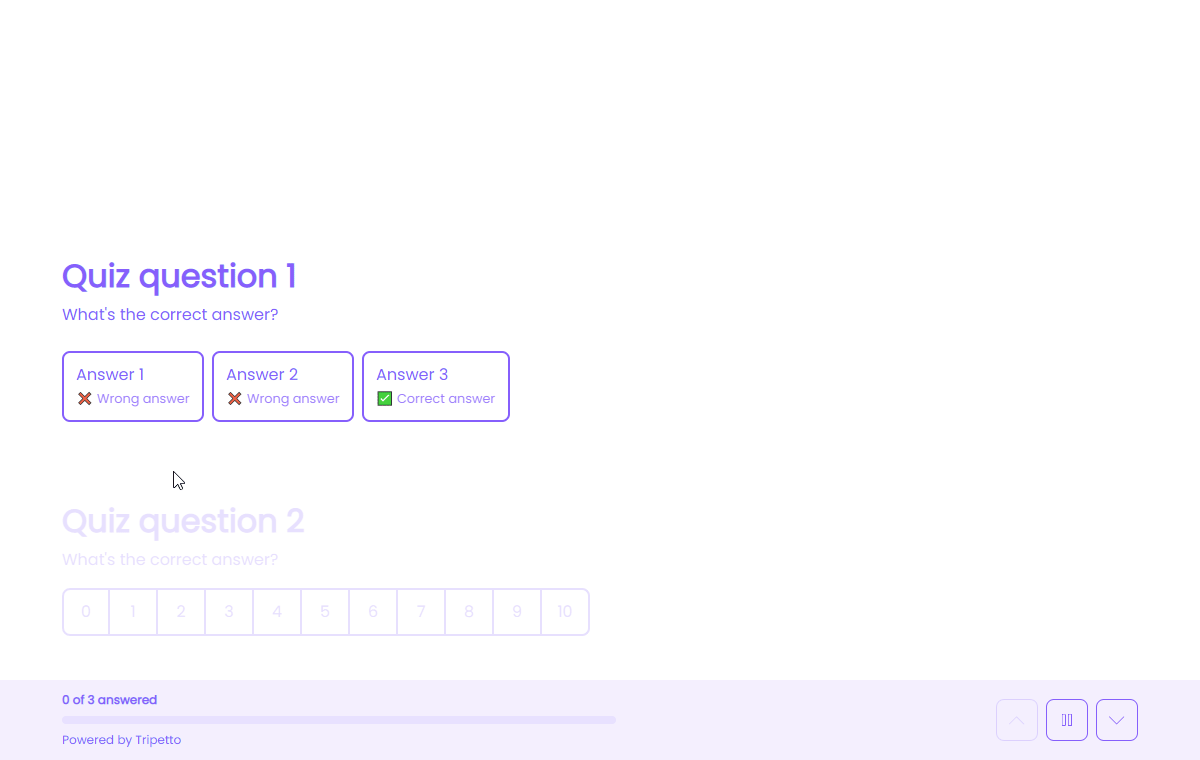Using logic3 Min.

# How to use the outcomes of calculator blocks

The outcome of each calculator block can be used in several ways inside your form and your dataset.

## When to use

Use the calculator block when you want to perform calculations inside your form. You can use the outcomes of calculator blocks in several ways, for example:

• Show the outcome of a calculator block to your respondent;
• Use the outcome of a calculator block as a branch condition;
• Use the outcome of a calculator block as the input for another calculator block;Demonstration of showing the quiz score and a different text based on the score.

## How to use

In the article you're reading now we describe how to use the outcomes of calculator blocks. For global instructions about the calculator block, please have a look at this article.

In general you can do the following with calculator outcomes:

• Save outcome in dataset;
• Show outcome in form (piping logic);
• Use outcome as input for other calculator block;
• Use outcome as value in other input block;
• Use outcome as logic condition (branch logic).

We will explain each of these possibilities below.

### Save outcome in dataset

By default the outcome of the calculator block will be saved to your dataset. That way the outcome will be available in your results, exports, notifications and webhooks.

### Show outcome in form (piping logic)

You can show the outcome of a calculator block to your respondent in the form in realtime. All calculator blocks are available for piping logic, by typing the `@` sign at the position you want to show the outcome.

### Use outcome as input for other calculator block

The outcome of each calculator block is also available in any calculator block that comes after. So you can use the outcome of the first calculator block as the input to a second calculator block.

### Use outcome as value in other input block

The outcome of each calculator block can also be used to set the value of other input blocks in your form, for example to update the value of a number input block.

### Use outcome as logic condition (branch logic)

You can use the outcome of a calculator block to check if a certain branch applies to your filter for that branch. The following filters are available for this:

• When calculation is equal to `your filter`;
• When calculation is not equal to `your filter`;
• When calculation is lower than`your filter`;
• When calculation is higher than `your filter`;
• When calculation is between `your filters`;
• When calculation is not between `your filters`;
• When calculation is valid;
• When calculation is not valid.

#### Filters

When we mention `your filter(s)` above, there are some different filters that you can use to make the right comparison:

• Number - Compare with a fixed number that you enter;
• Value - Compare with another block value supplied in the form by a respondent (more info).

The calculator block has lots of features, so we have several ways to learn all about it.

### Help center

Our help articles help you out on all different aspects of the calculator:

### Overviews

We also made some overviews of the capabilities that the calculator block provides: